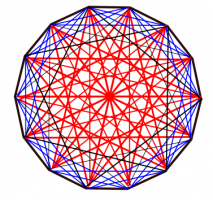# counting triangles

## Recommended Posts

there are N points on a paper. any three of points are not colinear.

you draw lines from each point to all other points.lines can be drawn with three colors; red,yellow or blue.

there will be obtained some triangles having colored edges.

purpose is not to get a triangle, edges of which are drawn with the same color.

what can be the value N at maximum?

##### Share on other sites

• 3 weeks later...

I think this problem is the same as arranging the points in a nice symmetric pattern; ie around the edge of circle - and it is much easier to visualize the relations

If you imagine numbering each point 1,2,..n around the circle then a triangle of a single colour is formed when you complete an entire circuit in three legs (ie from point 1 to point 4, point 7 and back to point 1 again). Alternatively you can see this triangle as a journey around in which the total steps add up to n. Example for seven points 1 to 2 to 4 to 1 is three legs making a triangle and the steps (1+2+4 = 7) add up to number of points. With point in a circle and always counting in same direction all triangle must have journey steps adding to total number of points.

It is quite easy to show that stars (and possibly a diagonal that halves the shape) that are made from moving a fixed number of steps around the circle will only make a triangle when (total number of points)/(step size) = 3. Similarly it is just a matter of adding three numbers to show that two stars overlaid will not make a triangle iff no three-step combination of their (step size) equals the (total number of points).

worked example 14 points

steps possible 1 (clockwise) = 13 (anticlock wise)

1,13

2,12

3,11

4,10

5,9

6,8

7,7

these can quite easily be divided into three groups/colours (7,7 6,8 5,9) (3,11 2,12) (4,11 1,13) within these groups no combination of 3 steps makes 14 - there are no triangles formed vertices at points and sides of same colour. Ie there is no 3-combination of 7,7 6,8 5,9 that equals 14, similarly with other two groups. Once all possible steps have been placed it is clear that every point is linked to every other point.

For all n from 3 through to 14 this works and at least one formation produces no triangles of one colour (with a little shenanigans for n=6,9,12). For n=15 this Does NOT work. I will continue to think and see if I can find a way to show that 15 is not possible in any method. I will also draw up a nice illustration.

Matthew - convinced that this is a dead question on a dead post , but still quite interested in itproof that 14 points can be linked (with links of 3 different colours) and no triangle with vertices at points is made of a single colour

Edited by imatfaal

## Create an account

Register a new account